## Linear Independence

Let A = { v 1, v 2, …, v r } be a collection of vectors from Rn . If r > 2 and at least one of the vectors in A can be written as a linear combination of the others, then A is said to be linearly dependent. The motivation for this description is simple: At least one of the vectors depends (linearly) on the others. On the other hand, if no vector in A is said to be a linearly independent set. It is also quite common to say that “the vectors are linearly dependent (or independent)” rather than “the set containing these vectors is linearly dependent (or independent).”

Example 1: Are the vectors v 1 = (2, 5, 3), v 2 = (1, 1, 1), and v 3 = (4, −2, 0) linearly independent?

If none of these vectors can be expressed as a linear combination of the other two, then the vectors are independent; otherwise, they are dependent. If, for example, v 3 were a linear combination of v 1 and v 2, then there would exist scalars k 1 and k 2 such that k v + k 2 v 2b = v 3. This equation reads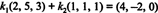which is equivalent toHowever, this is an inconsistent system. For instance, subtracting the first equation from the third yields k 1 = −4, and substituting this value into either the first or third equation gives k 2 = 12. However, (k 1, k 2) = (−4, 12) does not satisfy the second equation. The conclusion is that v 3 is not a linear combination of v 1 and v 2. A similar argument would show that v 1 is not a linear combination of v 2 and v 3 and that v 2 is nota linear combination of v 1 and v 3. Thus, these three vectors are indeed linearly independent.

An alternative—but entirely equivalent and often simpler—definition of linear independence reads as follows. A collection of vectors v 1, v 2, …, v r from R n is linearly independent if the only scalars that satisfy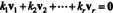are k 1 = k 2 = ⃛ = k r = 0. This is called the trivial linear combination. If, on the other hand, there exists a nontrivial linear combination that gives the zero vector, then the vectors are dependent.

Example 2: Use this second definition to show that the vectors from Example 1— v 1 = (2, 5, 3), v 2 = (1, 1, 1), and v 3 = (4, −2, 0)—are linearly independent.

These vectors are linearly independent if the only scalars that satisfy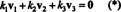are k 1 = k 2 = k 3 = 0. But (*) is equivalent to the homogeneous systemRow‐reducing the coefficient matrix yieldsThis echelon form of the matrix makes it easy to see that k 3 = 0, from which follow k 2 = 0 and k 1 = 0. Thus, equation (**)—and therefore (*)—is satisfied only by k 1 = k 2 = k 3 = 0, which proves that the given vectors are linearly independent.

Example 3: Are the vectors v 1 = (4, 1, −2), v 2 = (−3, 0, 1), and v 3 (1, −2, 1) linearly independent?

The equation k 1 v 1 + k 2 v 2 + k 3 v 3 = 0 is equivalent to the homogeneous systemRow‐reduction of the coefficient matrix produces a row of zeros: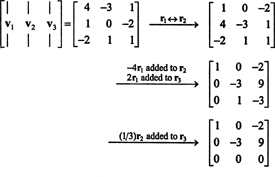Since the general solution will contain a free variable, the homogeneous system (*) has nontrivial solutions. This shows that there exists a nontrivial linear combination of the vectors v 1, v 2, and v 3 that give the zero vector: v 1, v 2, and v 3 are dependent.

Example 4: There is exactly one value of c such that the vectors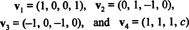are linearly dependent. Find this value of c and determine a nontrivial linear combination of these vectors that equals the zero vector.

As before, consider the homogeneous systemand perform the following elementary row operations on the coefficient matrix:In order to obtain nontrivial solutions, there must be at least one row of zeros in this echelon form of the matrix. If c is 0, this condition is satisfied. Since c = 0, the vector v 4 equals (1, 1, 1, 0). Now, to find a nontrivial linear combination of the vectors v 1, v 2, v 3, and v 4 that gives the zero vector, a particular nontrivial solution to the matrix equationis needed. From the row operations performed above, this equation is equivalent toThe last row implies that k 4 can be taken as a free variable; let k 4 = t. The third row then saysThe second row implies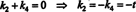and, finally, the first row gives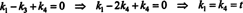Thus, the general solution of the homogeneous system (**)—and (*)—is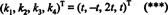for any t in R. Choosing t = 1 , for example, gives k 1, k 2, k 3, k 4 sois a linear combination of the vectors v 1, v 2, v 3, and v 4 that equals the zero vector. To verify that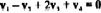simply substitute and simplify: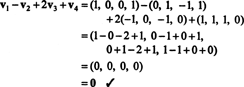Infinitely many other nontrivial linear combinations of v 1, v 2, v 3, and v 4 that equal the zero vector can be found by simply choosing any other nonzero value of t in (***) and substituting the resulting values of k 1, k 2, k 3, and k 4 in the expression k 1 v 1 + k 2 v 2 + k 3 v 3 + k 4 v 4.

If a collection of vectors from R n contains more than n vectors, the question of its linear independence is easily answered. If C = { v 1, v 2, …, v m } is a collection of vectors from R n and m > n, then C must be linearly dependent. To see why this is so, note that the equationis equivalent to the matrix equation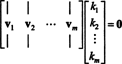Since each vector v j contains n components, this matrix equation describes a system with m unknowns and n equations. Any homogeneous system with more unknowns than equations has nontrivial solutions, a result which applies here since m > n. Because equation (*) has nontrivial solutons, the vectors in C cannot be independent.

Example 5: The collection of vectors {2 ij, i + j, − i + 4j} from R 2 is linearly dependent because any collection of 3 (or more) vectors from R 2 must be dependent. Similarly, the collection { i + jk, 2i3j + k, i4k, − 2j,− 5i + j3k} of vectors from R 3 cannot be independent, because any collection of 4 or more vectors from R 3 is dependent.

Example 6: Any collection of vectors from Rn that contains the zero vector is automatically dependent, for if { v 1, v 2,…, v r−1, 0} is such a collection, then for any k ≠ 0,is a nontrivial linear combination that gives the zero vector.